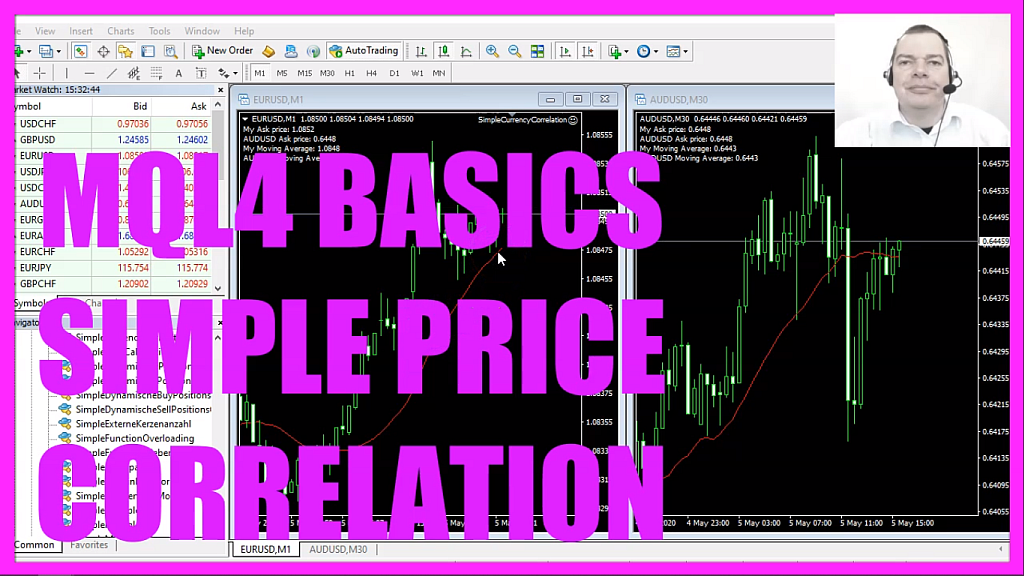In this video we are going to create an expert advisor that is able to calculate the values for a simple moving average for two currency pairs, so let’s find out how to do that with mql4.
To get started please click on a little icon here or press F4 on your keyboard. Now you should see the Metaeditor and here you want to click on file, new file, expert advisor from template, continue, I will call this file, simple currency correlation, click on continue, continue and finish.
Now you can delete everything above the ontick function and the two comment lines here.
We start by calculating the ask price, this is a little bit different, in mql4 it is possible to just use the ask price without calculating it but this is only possible if you want to know the ask price for the current symbol and this is not the case, so we use a backported solution from mql5 and here is how it works, we use a function that is called symbol info double, this function takes a few parameters, the first one is the current symbol on the chart, the second one is what we want to know, in this case, we want to know the ask price so we use symbol underscore ask – all in capital letters – and with normalize double and underscore digits we make sure to calculate the right number of digits behind the dot depending on the currency pair that might be three or five digits.
And that’s how we do it for the current ask price on the current chart but we want it also to calculate the ask price for another currency pair and here is how we do it, it’s almost the same except that we are using the currency pair as a hard-coded string variable for the first parameter here and of course we use a different name for the variable, please make sure to exactly spell the currency pair as it shows up on your Metatrader screen. Depending on the account type you use you might see a different, this is what it would look like in Metatrader 5 for my RoboForex account.
And now we have the ask price for the current currency pair and for the one that we want to compare.
Now let’s continue with the moving average.
To calculate it we use the integrated ima function for the current symbol on the chart, we use the period m one constant here because I want to know the values for the one-minute chart, I want to use twenty candles for the calculation, no shift value, it should be a simple moving average so we use mode underscore sma, the result should be calculated based on the close price for candle number zero, that’s the current candle on the current chart.
Now let’s repeat that for the second currency pair. It’s almost similar but this time we have also hard-coded the currency pair, please keep in mind that this is just about the concept, usually, you wouldn’t hard code anything but for this little video, it is good enough.
Now let’s create a chart output by using the comment statement, it will output the current ask price for the current currency pair, the ask price for the other currency pair, the moving average for the current chart and the moving average for the Australian against the US dollar.